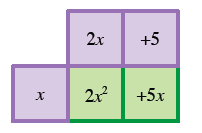### Home > PC3 > Chapter 1 > Lesson 1.1.6 > Problem1-58

1-58.

Factor each binomial.

1. $2x^2+5x$

Use a generic rectangle or look for common factors in each term.1. $3x^2y^5-9xy^3$

The two terms have a common factor of $3xy^3$.

1. $17x^3y-34x^4y^2$

$17x^{3}y(1-2xy)$